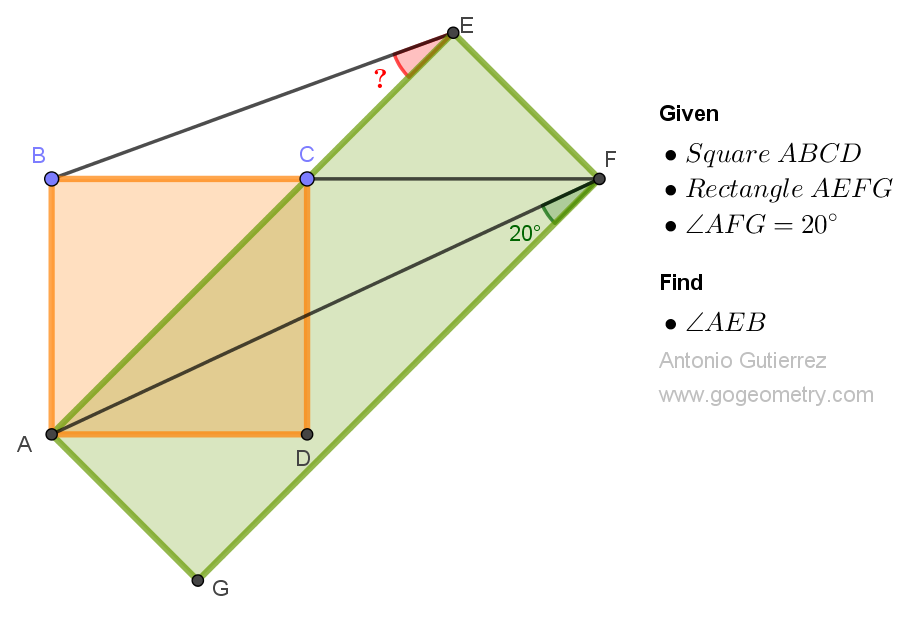## Tuesday, March 28, 2023

### Geometry Problem 1530: Unlock the Secrets of Geometric Angles: Calculate the Measurement of an Angle in a Square and Rectangle Figure Today

Geometry Problem 1530. Post your solution in the comment box below.
Level: Mathematics Education, K-12 School, Honors Geometry, College.

Details: Click on the figure below.More Details

To post a solution to this problem click Comment underneath the post, or click into the line that says, “Enter Comment.” Type what you want to say and press Publish to post your solution.

1.ABEF is a circle with AF as diameter.
So < BFE = < BAE = 45 and so
< AFB = 90 - 45 - 20 = 25

Therefore < AEB = < AFB = 25

Sumith Peiris
Moratuwa
Sri Lanka

2.25°

(20+45)+(90+AEB)=180
AEB=25

3.x=25° : https://photos.app.goo.gl/d7LNrJchKUm4RTG66

4.ABEF is a cyclic quadrilateral so ^(BEA)=^(BFA)
ABCD is a square => ^(BCA)=^(ECF)=^(EFC)= 45
so ^(BFA)=^(BEA)=90-45-20=25

5.Angle ABF = Angle AEF = 90 degrees, hence Quadrilateral ABEF is cyclic with AF as diameter.
Angle BAE= Angle BFE =45 deg.
Angle AFB= 90 - 45 - 20 = 25 deg.
Since ABEF is cyclic Angle AEB = Angle AFB = 25 degrees.

6.